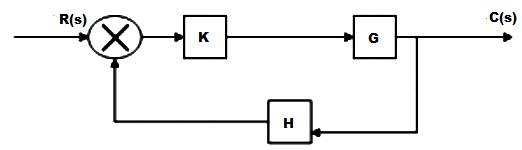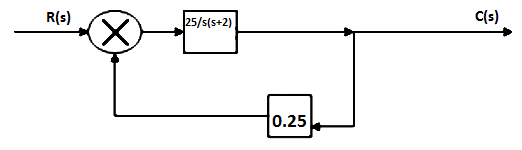# Feedback Compensation

Feedback Compensation

1. Which of the following are the not characteristics of the closed loop systems?

a) It does not compensate for disturbance
b) It reduces the sensitivity of plant-parameter variations
c) It does not involve output measurements
d) It does not has the ability to control the system transient response

Explanation: Feedback refers to the comparison of the final output to the desired output at respective input so as to get accurate and error free result and in the system improves the transient response of the system.

2. Which one of the following effect in the system is not caused by negative feedback?

a) Reduction in gain
b) Increased in bandwidth
c) Increase in distortion
d) Reduction in output impedance

Explanation: Distortion refers to the error in the open loop system and it has many oscillations in the output and is reduced in case of negative feedback.

3. Which of the statement is correct with regard to the bandwidth of the control loop system:

a) In systems where the low frequency magnitude in 0 dB on the bode diagram, the bandwidth is measured at the -3 dB frequency
b) The bandwidth is the measurement of the accuracy of the closed loop system
c) The stability is proportional to the bandwidth
d) The system with larger bandwidth provides slower step response and lower fidelity ramp response

Explanation: Bandwidth is the frequency measured at the gain of 3dB and for the good control system the value of the bandwidth must be large but the large value of the bandwidth increses the noise in the system.

4. Which of the statements are the advantages of closed loop systems over the open loop system:

a) The overall reliability of the open loop system is more than the closed loop system is more than closed loop system.
b) The transient response in closed loop system decays more quickly than in open loop system.
c) In open loop system closing the loop increases the gain of the system
d) In open loop system the effect of parameter variation is reduced

Explanation: Speed of response refers to the time taken to give the final output and it depends upon the rise and settling time of the transient response and speed of response of closed loop system is more than that of open loop system.

5. In control system excessive bandwidth is not employed because

a) Noise is proportional to bandwidth
b) It leads to low relative stability
c) It leads to slower time response
d) Noise is proportional to the square of the bandwidth

Explanation: In closed loop system the bandwidth of the system is more as compared to the open loop system and this is so required as higher the bandwidth means lower the selectivity and hence higher the noise.

6. Feedback control system is basically

a) High pass filter
b) Low pass filter
c) Band pass filter
d) Band stop filter

Explanation: Low pass filter is mainly as integral controller and it is used as the controller in the system so as to increase the accuracy by reducing or proper eliminating the steady state error of the control system.

7. Which of the following will not decrease as a result of introduction of negative feedback?

a) Instability
b) Bandwidth
c) Overall gain
d) Distortion

Explanation: Bandwidth always increases due to negative feedback as the speed of response is directly proportional to the bandwidth.

8. As compared to the closed loop system, an open loop system is

a) More stable but less accurate
b) Less stable as well as less accurate
c) More stable as well as more accurate
d) Less stable but more accurate

Explanation: Open loop system always follows the input but closed loop system always reduces the error irrespective of the input applied.

9. Insertion of negative feedback in control system affects:

a) The transient response to vanish uniformly
b) The transient response to decay very fast
c) No change in transient response
d) The transient response decays at slow rate

Explanation: Feedback can be positive or negative but practically positive feedback is not used as it causes oscillations in the system with more gain and hence negative feedback is use which causes speed of response to increase.

10. Open loop system is ___________ stable than closed loop system

a) More
b) Less
c) Inclined
d) Exponential

Explanation: Open loop system always follows the input but closed loop system always reduces the error irrespective of the input applied.

11. For the gain feedback system, does not affect the system output if KG is :a) Small
b) Negative
c) One
d) Very large

Explanation: T(s)=KG/(1+KGH)
If KG>>1: then C(s)=R(s)/H.

12. Determine the sensitivity of the overall transfer function for the system shown in the figure below, at w=1 rad/sec with respect to the feedback path transfer function.a) 1.11
b) -1.11
c) 2.22
d) -2.22

Explanation: S= -G(s)H(s)/1+G(s)H(s)-1.1.

13. Primary purpose of using Feedback is :

a) To reduce the sensitivity of the system to parameter variations.
b) To increase the bandwidth of the system
c) To reduce the noise and distortion of the system
d) To increase stability of the system